# 以线性回归为例说明指标加速的 3 种方法

31 十二月 2013, 10:090
2 297

### 基本指标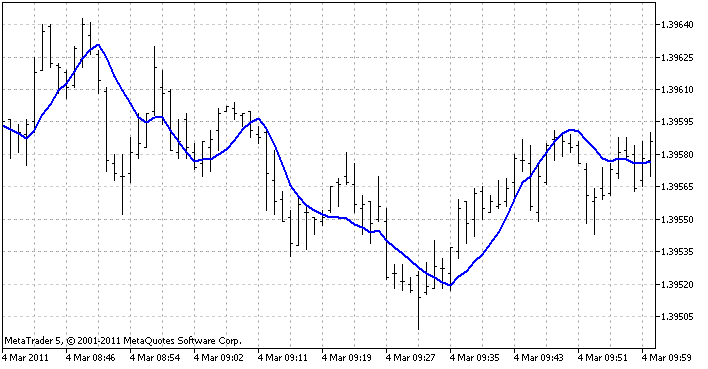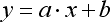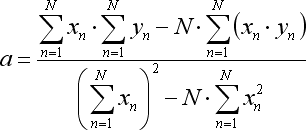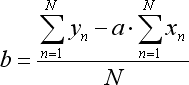```            // Finding intermediate values-sums
Sx  = 0;
Sy  = 0;
Sxx = 0;
Sxy = 0;
for (int x = 1; x <= LRPeriod; x++)
{
double y = price[bar-LRPeriod+x];
Sx  += x;
Sy  += y;
Sxx += x*x;
Sxy += x*y;
}

// Regression ratios
double a = (LRPeriod * Sxy - Sx * Sy) / (LRPeriod * Sxx - Sx * Sx);
double b = (Sy - a * Sx) / LRPeriod;

lrvalue = a*LRPeriod + b;```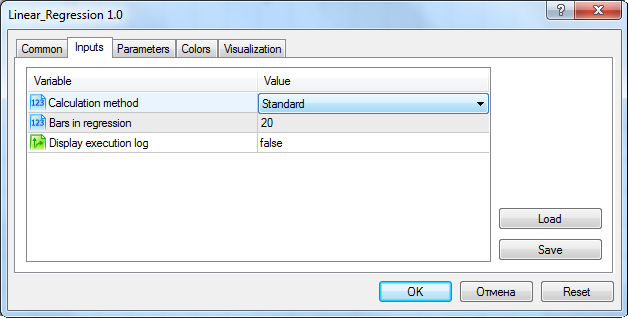### 第一种优化方法。移动总和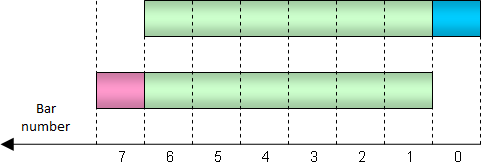`double ExtBufSx[], ExtBufSy[], ExtBufSxx[], ExtBufSxy[];`

```   SetIndexBuffer(1, ExtBufSx,  INDICATOR_CALCULATIONS);
SetIndexBuffer(2, ExtBufSy,  INDICATOR_CALCULATIONS);
SetIndexBuffer(3, ExtBufSxx, INDICATOR_CALCULATIONS);
SetIndexBuffer(4, ExtBufSxy, INDICATOR_CALCULATIONS);```

```            // (The very first bar was calculated using the standard method)

// Previous bar
int prevbar = bar-1;

//--- Calculating new values of intermediate totals
//    from the previous bar values

Sx  = ExtBufSx [prevbar];

// An old price comes out, a new one comes in
Sy  = ExtBufSy [prevbar] - price[bar-LRPeriod] + price[bar];

Sxx = ExtBufSxx[prevbar];

// All the old prices come out once, a new one comes in with an appropriate weight
Sxy = ExtBufSxy[prevbar] - ExtBufSy[prevbar] + price[bar]*LRPeriod;

//---

// Regression ratios (calculated the same way as in the standard method)
double a = (LRPeriod * Sxy - Sx * Sy) / (LRPeriod * Sxx - Sx * Sx);
double b = (Sy - a * Sx) / LRPeriod;

lrvalue = a*LRPeriod + b;```

### 第二种方法。简化

• 总和 y 以简单移动平均表示：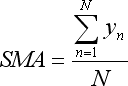• 总和 x*y 以线性加权移动平均表示：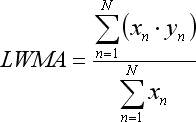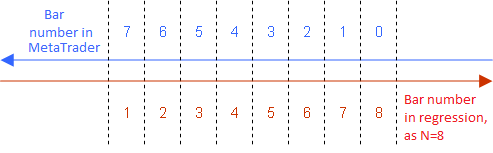• x 总和是 (1 + 2 + ...+ N) 数字序列的总和，可被以下等式代替：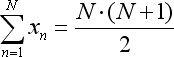• x*x 总和依据另一等式简化：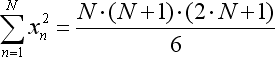• 要建立一个指标图表，我们只需要针对 x ＝ N 的最后一根柱计算回归函数的平均值，即回归函数等式可被其具体情形代替：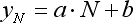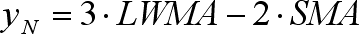```            double SMA ;
double LWMA;
CopyBuffer(h_SMA,  0, rates_total-bar, 1, SMA);
CopyBuffer(h_LWMA, 0, rates_total-bar, 1, LWMA);

lrvalue = 3*LWMA - 2*SMA;```

LWMA 和 SMA 指标是在 OnInit 中初始创建的：

```      h_SMA  = iMA(NULL, 0, LRPeriod, 0, MODE_SMA,  PRICE_CLOSE);
h_LWMA = iMA(NULL, 0, LRPeriod, 0, MODE_LWMA, PRICE_CLOSE);```

### 第三种方法。近似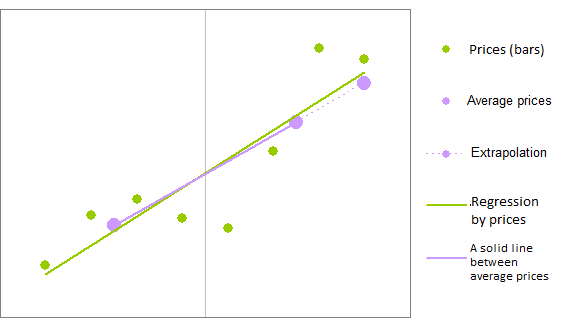```           // The interval midpoint
int HalfPeriod = (int) MathRound(LRPeriod/2);

// Average price of the first half
double s1 = 0;
for (int i = 0; i < HalfPeriod; i++)
s1 += price[bar-i];
s1 /= HalfPeriod;

// Average price of the second half
double s2 = 0;
for (int i = HalfPeriod; i < LRPeriod; i++)
s2 += price[bar-i];
s2 /= (LRPeriod-HalfPeriod);

// Price excess by one bar
double k = (s1-s2)/(LRPeriod/2);

// Extrapolated price at the last bar
lrvalue = s1 + k * (HalfPeriod-1)/2;```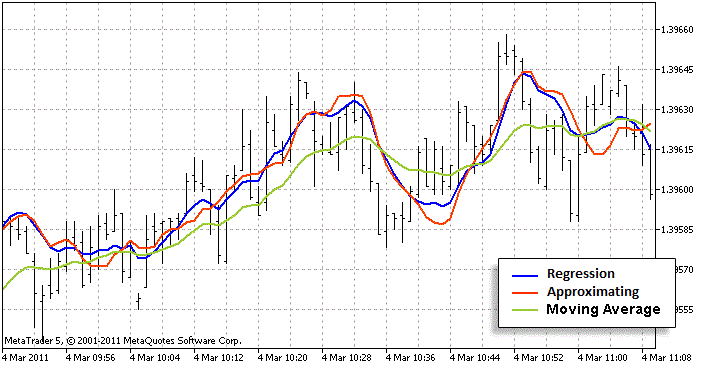红线比绿线更接近蓝线。这意味着近似算法很好

### 速度比较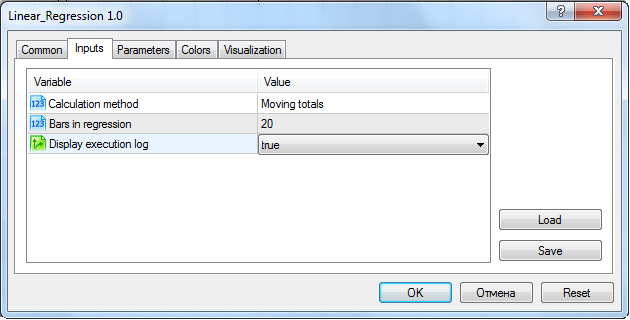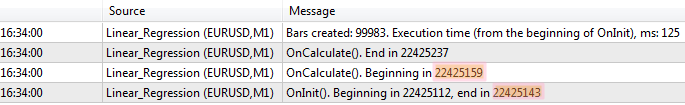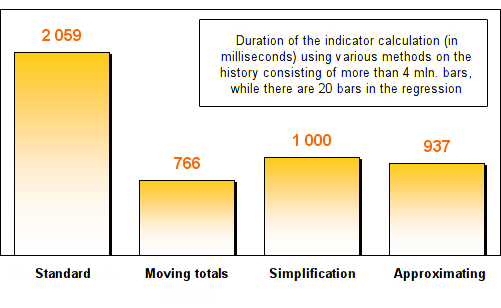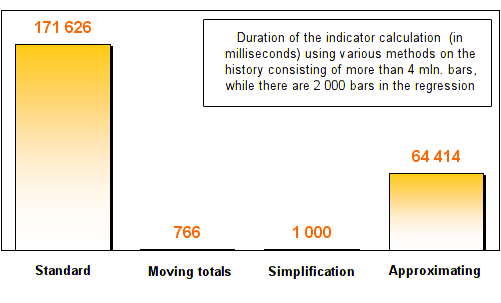### 总结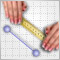统计分布在交易者工作中的作用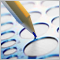使用伪模板替代 C++ 模板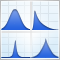用 MQL5 表示统计概率分布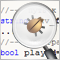源代码的跟踪、调试和结构分析